# GED Math : Operations with Negative Numbers

## Example Questions

### Example Question #21 : Operations With Negative Numbers

An airplane takes off from Death Valley, which is 282 feet below sea level. It ascends 12,879 feet before leveling off, then it ascends 4,276 feet; it finally descends 1,874 feet. Which of the following expressions is equal to the plane's current elevation in feet?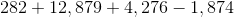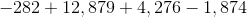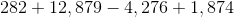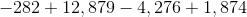Explanation:

The initial elevation of the plane is 282 feet below sea level, which is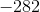feet in elevation. Ascent is an increase in elevation and descent is a decrease, so, to the elevation, in turn, add 12,879 feet, add 4,276 feet, and subtract 1,874 feet.

This expression is.

### Example Question #22 : Operations With Negative Numbers

How many of these statements are correct?

I)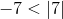II)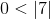III)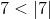None

Two

One

Three

Two

Explanation:

The absolute value of positive number is the number itself, so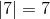. Of the three numbers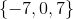, 7 is not less than 7 itself, but the other two are.

### Example Question #23 : Operations With Negative Numbers

Evaluate: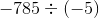Do not use a calculator.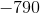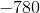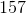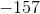Explanation:

To divide two numbers of like sign, just divide their absolute values; their quotient will be positive.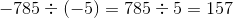### Example Question #21 : Operations With Negative Numbers

Solve: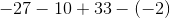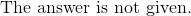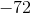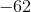Explanation:

When a negative number is subtracted with a positive number, we will have an increasingly negative number.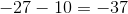Double negative signs will translate into a positive sign.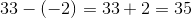Sum the two numbers.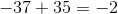The answer is:### Example Question #25 : Operations With Negative Numbers

Solve: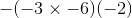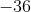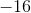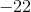Explanation:

Evaluate the terms in the parentheses first.  Double negatives will turn into a positive.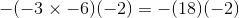Simplify this expression.

The answer is:### Example Question #26 : Operations With Negative Numbers

Evaluate: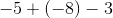Explanation:

A positive and a negative sign will be combined as a negative.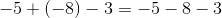When a negative number is subtracted, it becomes increasingly negative.

The answer is:### Example Question #27 : Operations With Negative Numbers

Evaluate: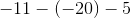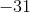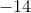Explanation:

Double negatives can be converted to a positive sign.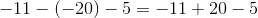Compute the sum.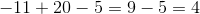The answer is:### Example Question #23 : Operations With Negative Numbers

Simplify the following: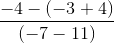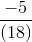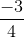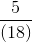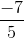Explanation:

Begin by simplifying everything that is found in parentheses: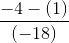Next, perform the subtraction in the numerator: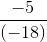Although you cannot simplify the fraction, you can cancel out the negatives since you have a negative in both the numerator and the denominator.  Thus, your answer is:### Example Question #22 : Operations With Negative Numbers

Simplify the following: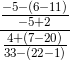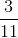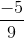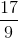Explanation:

Okay, this is complex.  However, just do it step by step and you will surely get it correct!  First, handle everything in parentheses: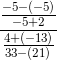Next, simplify each of the smaller fractions: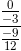Now, remember that to divide two fractions, you need to multiply by the reciprocal, this means: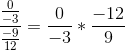You don't have to do any more work, however!  The answer is, of all things,!  This is because the first fraction is equal to.

### Example Question #30 : Operations With Negative Numbers

Subtract: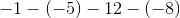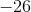Explanation:

Convert double negatives to a positive sign.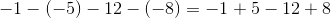The answer is: